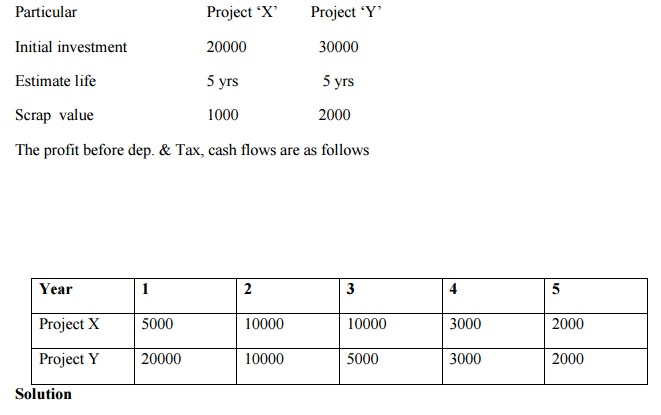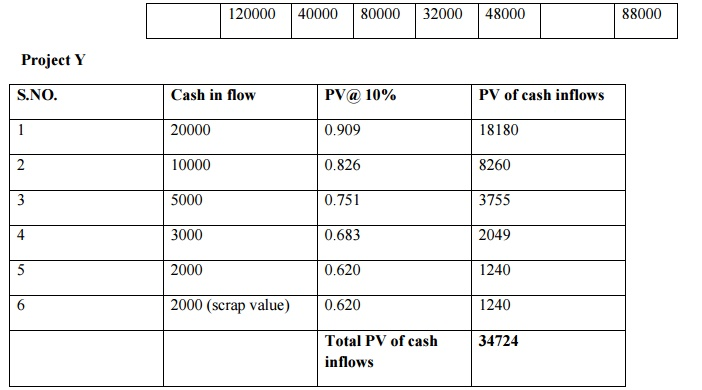Home | | Engineering Economics and Financial Accounting | Evaluation of Investment Proposals

# Evaluation of Investment Proposals

Evaluation Of Investment Proposals

·                    8.2.1Net Present Value method

·              Internal Rate of Return method

·              Rate of return method or accounting method

(i) Net Present Value method

(ii) Internal Rate of Return method

(iii)Profitability Index method

1. Pay-back period method

This method represent the period in which total investment in permanent asset pays back itself. It measure the period of time for the original cost of a project to be recovered from the additional earning of a project itself.

Investment are ranked according to the length of the payback period, investment with shorter payback period is preferred.

How the payback period is calculated?

The payback period is ascertained in the following manner

·                    Calculate annual net earnings(profit) before depreciation and after taxes, these are called annual cash inflow

·                    Divide the initial outlay(cost) of the project by the annual cash inflow, where the project generates constant annual cash inflow

Payback period = cash outlay of the project or original cost of the asset / Annual cash inflows

·        Where the annual cash inflows (profit before depreciation and after taxes) are unequal the payback period is found by adding up the cash inflows until the total is equal to the initial cash outlay of the project.

Selection criterionImprovement of Traditional Appraoch To Payback Period:Post payback profitability =  Annual cash inflow (estimated life-payback period)

Payback Reciprocal MethodDiscounted payback period : - (time value of money in consider)

Merits

v   It is a simple method to calculate and understand

v   It is a method in terms of years for easier appraisal

Demerits

v   It is a method rigid

v   It has completely discarded the principle of time value of money

v   It has not given any due weight age to cash inflows after the payback period

v   It has sidelined the profitability of the project.

2. Average Rate of Return method (ARR)

This method takes in to account the earnings expected from the investment over their whole life. It is known as accounting rate of return.

The project which gives the higher rate of return is selected when compared to one with lower rate of

return.

Selection criterion of the projects:Merits

v   It is simple method to compute the rate of return

v   Average return is calculated from the total earnings of the enterprise through out the life of the firm

v   The entire rate of return is being computed on the basis of the available accounting data

Demerits

v   Under this method, the rate of return is calculated on the basis of profits extracted from the books but not on the basis of cash inflows

v   The time value of money is not considered

v   It does not consider the life period of the project

v   The accounting profits are different from one concept to another which leads to greater confusion in determining the accounting rate of return of the projects

3. Net present value method(NPV)

It is a modern method of evaluating investment proposals. It takes into consideration time value of money and calculates the return on investment by introducing the factor of time element.

v   First determine the rate of interest that should be selected as the minimum required rate of return

v   Compute the present value of total investment outlay

v   Compute the present value of total cash inflows

v   Calculate Net Present Value by subtracting the present value of cash inflow by present value of cash outflow.

v   NPV = is positive or zero the project is accepted

v   NPV= is negative then reject the proposal

v   In order for ranking the project the first preference is given to project having maximum positive net present value

NPV= Present value of cash inflow – present value of cash outflow/Initial investment

Selection criterion of Net present value method4. Internal Rate of Return Method (IRR)

Under the internal rate of return method, the cash flows of a project are discounted at a suitable rate by hit and trial method, which equates the net present value so calculated to the amount of investment.

v   Determine the future net cash flows during the entire economic life of the project. The cash inflows are estimated for future profits before depreciation but after taxes

v   Determine the rate of discount at which the value of cash inflow is equal to the present value of cash outflows

v   Accept the proposal if the internal rate of return is higher than or equal to the cost of capital or cut off rate.

v   In case of alternative proposals select the proposal with the highest rate of return.

5. Profitability index method or Benefit cost Ratio (P.I)

It is also called Benefit cost ratio is the relationship between present value of cash inflow and present value of cash outflowThe proposal is accepted if the profitability index is more than one and is rejected the profitability index is less than one

The various projects are ranked; the project with higher profitability index is ranked higher than other.

1. Profitability Index Method (or) Benefit cost Ratio: -1. Calculate the average rate of return for Projects X and Y from the followingv   If the required rate of return is 10% which project should be undertaken?

v   Average Rate of Return = Original Investment / Average Annual Income X100

v   The first step is to find out the average annual income of the two different projects X and Y

v   Average Annual Income Total income throughout the Project / Life of the Project

v   Average Annual Income (Project X) = Rs. 12,000 / 4 years = Rs. 3,000

v   Average Annual Income (Project Y) = Rs. 20,000 / 5 years = Rs. 4,000

v   The next step is to find out the Average rate of return:

v   Average rate of return (Project X) = Rs. 3,000 / Rs.40,000 X100 =7.5%

v   Average rate of return (Project Y) = Rs.5,000/  Rs. 60,000 X 100 = 8.33%

v   Both the projects are lesser than the given required rate of return. These two projects are not advisable to invest only due to lesser accounting rate of return.

2. Calculate the NPV of 2 projects and suggest which of 2 projects should be accepted assuming a discount rate 10%NPV = PV cash of inflow  - PV of cash outflows

= 24224 - 20000 NPV = 4224NPV = PV of cash inflow        - PV of cash outflows

NPV = 34724 – 30000

NPV = 4724

Comment:

NPV of project y is higher than the NPV of project x. Hence, it is suggested that project y should be selected.

3. Initial outlay Rs. 50000, life of an asset 5 years Annual cash flow Rs. 12500, Calculate IRRPresent value of annuity table 8 % approximately

IRR = 8 %

Illustration

When the annual cash flows over the life of the asset.

Initial investment Rs. 60000, Life of the Assets 4 years

1st year - 15000

2nd year – 20000

3rd year – 30000

4th year -20000

Calculate the IRRStudy Material, Lecturing Notes, Assignment, Reference, Wiki description explanation, brief detail
Engineering Economics and Financial Accounting : Investments : Evaluation of Investment Proposals |